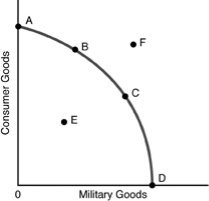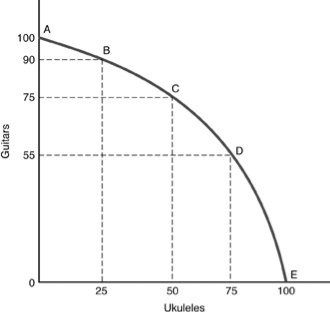• FAQ
• Contact/ Homework Answers / Economics / 81) Which of the following assumed when constructing a production
Not my Question
Flag Content

# Question : 81) Which of the following assumed when constructing a production

81) Which of the following is assumed when constructing a production possibilities curve?

A) a fixed amount of resources

B) the efficient use of resources

C) resources of a given quality

D) All of the above are correct.

82) Which of the following is held constant when constructing a production possibilities curve?

A) the price level

B) the income level

C) combination of goods produced

D) the amount of total resources used

83) In constructing a production possibilities curve, all of the following are assumed EXCEPT

A) resources are fully employed.

B) the quantity and quality of resources being used is fixed.

C) the state of technology is improving.

D) the time period involved is fixed.

84) Inefficient use of resources is shown on the production possibilities curve

A) by an inward shifting of the curve.

B) by a point inside the curve.

C) by a point near the top of the curve.

D) by a point outside the curve.

85) A bowed-outward production possibilities curve demonstrates the concept of

A) constant opportunity costs as production shifts from the production of one good to the production of the other good.

B) decreasing opportunity costs as production shifts from the production of one good to the production of the other good.

C) increasing opportunity costs as production shifts from the production of one good to the production of the other good.

D) increasing opportunity costs at first but the opportunity costs steadily decrease as you move down along the curve.

86) The production possibilities curve for two products is bowed out because

A) as the production of a good increases, opportunity cost increases.

B) as the production of a good increases, opportunity cost is unchanged.

C) the qualities of the resources are not identical.

D) there are unemployed resources.87) Refer to the above figure. Production at Point F

A) is not attainable given the underlying assumptions of the production possibilities curve (PPC).

B) would not be desirable.

C) can only be attained by giving up Point E.

D) can be attained only if a society desired more goods and services.

88) Refer to the above figure. Production at Point E

A) would indicate that this economy is producing beyond its capabilities.

B) would indicate production at a level below that which is attainable.

C) is not attainable with given resources and technology.

D) would demonstrate a total lack of technical expertise.

89) When a society takes increasing amounts of resources and applies them to the production of a specific good, resulting in increasing opportunity costs for each additional unit produced, which of the following applies?

A) the law of demand

B) the law of supply

C) the law of scarcity

D) the law of increasing additional costs

90) Efficiency is achieved

A) when output is being produced at a point inside a production possibilities curve.

B) when producers are getting the maximum possible output from the available resources.

C) when consumers are able to buy everything that they want.

D) when prices of all goods and services go to zero.## Solution 5 (1 Ratings )

Solved
Economics 4 Months Ago 3 Views